# Fourier series in orthogonal polynomials

(diff) ← Older revision | Latest revision (diff) | Newer revision → (diff)

A series of the form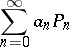(1)

where the polynomials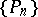are orthonormal on an intervalwith weight function(see Orthogonal polynomials) and the coefficientsare calculated from the formula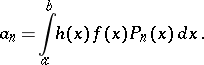(2)

Here, the functionbelongs to the class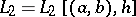of functions that are square summable (Lebesgue integrable) with weight functionover the interval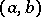of orthogonality.

As for any orthogonal series, the partial sums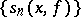of (1) are the best-possible approximations toin the metric of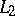and satisfy the condition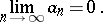(3)

For a proof of the convergence of the series (1) at a single pointor on a certain set inone usually applies the equality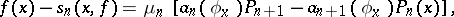where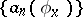are the Fourier coefficients of an auxiliary function, given by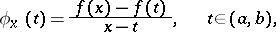for fixed, and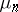is the coefficient given by the Christoffel–Darboux formula. If the interval of orthogonalityis bounded, if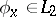and if the sequenceis bounded at the given point, then the series (1) converges to the value.

The coefficients (2) can also be defined for a functionin the class, that is, for functions that are summable with weight functionover. For a bounded interval, condition (3) holds if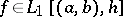and if the sequenceis uniformly bounded on the whole interval. Under these conditions the series (1) converges at a certain point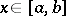to the valueif.

Letbe a part ofon which the sequenceis uniformly bounded, let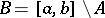and letbe the class of functions that are-summable overwith weight function. If, for a fixed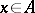, one has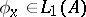and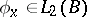, then the series (1) converges to.

For the series (1) the localization principle for conditions of convergence holds: If two functionsandincoincide in an interval, where, then the Fourier series of these two functions in the orthogonal polynomials converge or diverge simultaneously at. An analogous assertion is valid ifandbelong to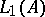and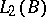and.

For the classical orthogonal polynomials the theorems on the equiconvergence with a certain associated trigonometric Fourier series hold for the series (1) (see Equiconvergent series).

Uniform convergence of the series (1) over the whole bounded interval of orthogonality, or over part of it, is usually investigated using the Lebesgue inequalitywhere the Lebesgue function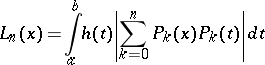does not depend onandis the best uniform approximation (cf. Best approximation) to the continuous functiononby polynomials of degree not exceeding. The sequence of Lebesgue functions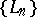can grow at various rates at the various points of, depending on the properties of. However, for the whole intervalone introduces the Lebesgue constantswhich increase unboundedly as(for different systems of orthogonal polynomials the Lebesgue constants can increase at different rates). The Lebesgue inequality implies that if the condition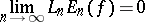is satisfied, then the series (1) converges uniformly toon the whole interval. On the other hand, the rate at which the sequence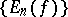tends to zero depends on the differentiability properties of. Thus, in many cases it is not difficult to formulate sufficient conditions for the right-hand side of the Lebesgue inequality to tend to zero as(see, for example, Legendre polynomials; Chebyshev polynomials; Jacobi polynomials). In the general case of an arbitrary weight function one can obtain specific results if one knows asymptotic formulas or bounds for the orthogonal polynomials under consideration.

How to Cite This Entry:
Fourier series in orthogonal polynomials. Encyclopedia of Mathematics. URL: http://encyclopediaofmath.org/index.php?title=Fourier_series_in_orthogonal_polynomials&oldid=13878
This article was adapted from an original article by P.K. Suetin (originator), which appeared in Encyclopedia of Mathematics - ISBN 1402006098. See original article